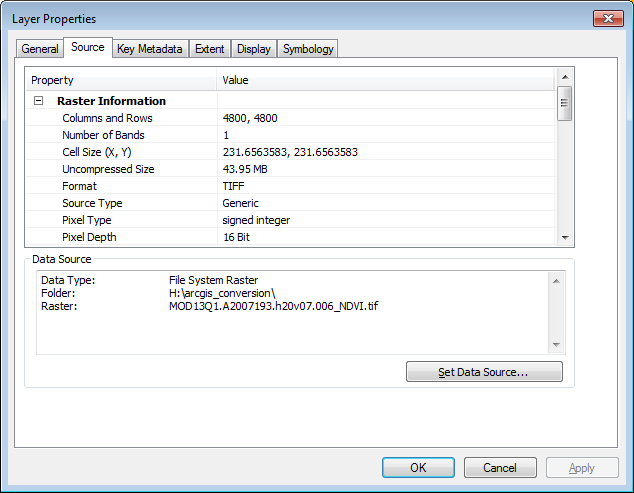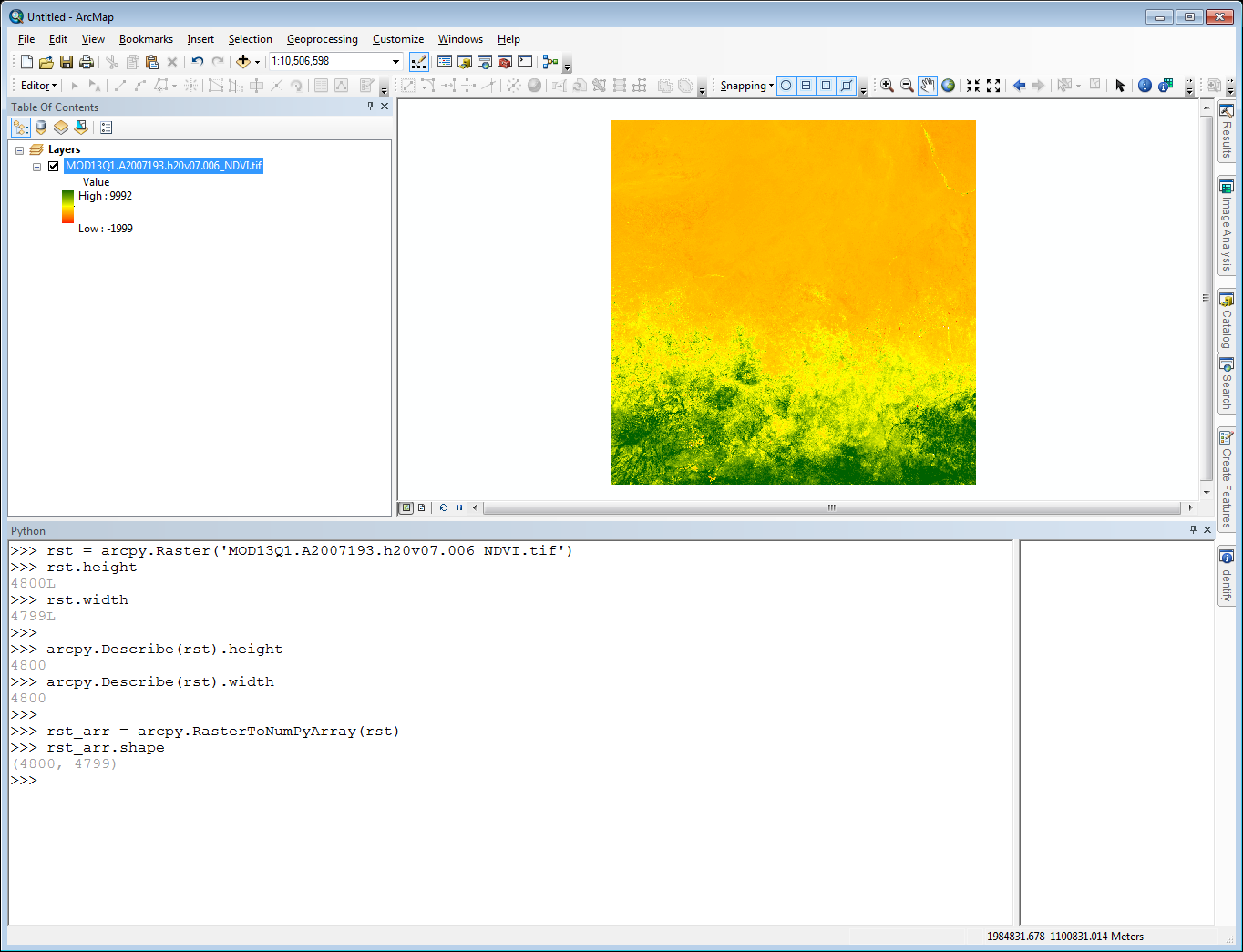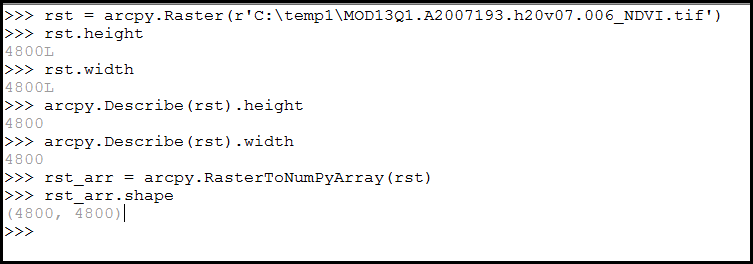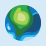# Arcpy RasterToNumPyArray Conversion Problem

2393
8
04-05-2017 02:03 PMNew Contributor II

I am executing arcpy commands from the python window in ArcMap 10.41. I am creating an arcpy Raster object using a raster layer from the table of contents. I want to then convert the raster object to a numpy array object so that I can run it through some scripted routines resulting in a raster output. With that said, I've noticed some odd behavior when creating the arcpy Raster object which seems to impact the creation of the numpy array object.

The properties for the input raster layer are below. Note that the number of columns and rows is 4800 each.After creating the raster object in the python window, I took a look at the height (rst.height) and width (rst.width) properties. To my surprise, they did not match. However, when I execute 'arcpy.Describe(rst).height' and 'arcpy.Describe(rst).width' I get 4800 for both (See image below). Can anyone explain this behavior?Additionally, when I convert the raster object to a numpy array is get the dimensions (4800, 4799) which then carries over into any raster I make.

In the case of this raster layer, I'm losing the far right column during the conversions. In some over cases, I'm losing the bottom row of the raster layer. Initially I assumed the problem had something to do with the precision of the lat/lon values in the lower-left corner. After adjusting those I was able to get the correct number of rows and columns, but the data for the row or column that was missing initially was filled with nodata values.

Has anyone experienced and/or solved this before?

Tags (3)
1 Solution

Accepted SolutionsNew Contributor II

After some back and forth, Neeraj was able to replicate the behavior and concluded that the problem is a bug in the software.

For now a simple workaround is as follows:

• In the first step, when creating a raster object, point directly to the raster dataset instead of a raster layer that is added to your map.
• For example, If you had this dataset in a folder, say ‘C:\temp1’, you would do sometime like,• This should preserve all the rows and columns in the original raster.
8 RepliesbyMVP Esteemed Contributor

I think the issue is with the use of long/lat data.  As an intermediate test, you could try to convert out to an esri grid and try converting it to the numpy array.  I have produced much larger arrays, but I don't normally use tif files nor data which are in geographic coordinates.  The truncation could simply be floating point issues with the calculation of rows and columns.  If it is really an issue, then you could always produce an empty array or an array of zeros with the appropriate shape and 'add' your raster into it.. but that would be overkill for an extra row, when you can simply truncate your other arrays or set the extent differently

``````z= np.zeros((4,3), dtype='int')

a = np.arange(9).reshape(3,3)

z[:3,:] = a

z
Out:
array([[0, 1, 2],
[3, 4, 5],
[6, 7, 8],
[0, 0, 0]])‍‍‍‍‍‍‍‍‍‍‍‍``````New Contributor II

I am converting the raster to a numpy array using the arcpy's RasterToNumpyArray function. During that conversion is where I lose the row and the data it contains. At the moment I am restricted to using arcpy and numpy to do the conversion, and I don't want to add any more dependencies to ArcGIS tool I'm developing. Is there a way to add the raster data values to an empty array without using the RasterToNumpyArray function?

I did just notice something with the arcpy raster object...when I run the command 'rst.width'  I get '4799L'. However, if I pick a point from column 4800 from the image in my display and request the cell value for that point from my raster object (rst), I get the correct data value in return. So, while the raster object properties says it's of length 4799, the object still contains data values for column 4800. However those data values are not being transfer into the numpy array.New Contributor III

I have been having a similar issue. I posted about it on the gis stack exchange.

I have recently been having some issues converting rasters to numpy arrays. The RasterToNumPyArray tool seems to be adding or subtracting rows or columns depending on which dataset I'm working on.

For instance I have a raster that contains 114 rows, and 160 columns. After converting it to an array it has 115 rows, and 160 column. If I convert this array back to a raster the data appears to be shifted up.

I have tried specifying the number of rows and columns to process in the RasterToNumPyArray tool. The row and column count issue is fixed but the output raster is still shifted up one row and the top row of data gets deleted.

This issue happens whether the data is in a geographic or projected coordinate system. Any idea why this would be happening? I am including a python script where I was seeing this issue.

``````import arcpy
import numpy as np

from arcpy import env
from os import path

env.overwriteOutput = True
env.outputCoordinateSystem = arcpy.SpatialReference(4326)

input_ras = r'PATH_TO_INPUT_RASTER'
input_ras_name = path.basename(input_ras)

ras_desc = arcpy.Describe(input_ras)
rows = ras_desc.height
cols = ras_desc.width
x_cell_size = ras_desc.meanCellWidth
y_cell_size = ras_desc.meanCellHeight

lower_left_corner = arcpy.Point(ras_desc.extent.XMin, ras_desc.extent.YMin)

print input_ras_name
print 'rows:', rows
print 'cols:', cols
print lower_left_corner

arr = arcpy.RasterToNumPyArray(input_ras, lower_left_corner, cols, rows, -9999)
print '\narry based on input raster'
print 'rows:', np.shape(arr)
print 'cols:', np.shape(arr)

print '\nconverting array back to a raster'

output_ras = arcpy.NumPyArrayToRaster(arr, lower_left_corner, input_ras, input_ras)
output_ras.save(r'PATH_TO_OUTPUT_RASTER')‍‍‍‍‍‍‍‍‍‍‍‍‍‍‍‍‍‍‍‍‍‍‍‍‍‍‍‍‍‍‍‍‍‍``````

I also explored the row and column count a little more. The attached screen shot shows the row and column count three different ways - one of which is different. Could this mean anything?

The problem does not seem to occur after converting my tif to a GRID and then processing the data. Ideally though I would not want to perform this intermediate step.byMVP Esteemed Contributor

It is the tif thing then, I never have any problems with esri gridsNew Contributor III

Hi Stephen,

From what you describe, sounds like your issue is slightly different to Aaron's with a shift in the raster dataset when you convert it from a NumPyArray back to a raster.

I'd like to take a look and see if I can help you resolve this issue. Could you please send me a sample dataset where you are seeing this behavior? If the data isn't too large, you can zip and email it to me at
NRajasekar@esri.com. If the data is too large for email, shoot me an email anyway and I can send you instructions to upload the data to a cloud storage site.

Thanks,
NeerajNew Contributor III

Hi Aaron,

I'd like to take a look and see if I can help you resolve this issue. Could you please send me a sample dataset where you are seeing this behavior? If the data isn't too large, you can zip and email it to me at NRajasekar@esri.com . If the data is too large for email, shoot me an email anyway and I can send you instructions to upload the data to a cloud storage site.

Thanks,
NeerajNew Contributor II

After some back and forth, Neeraj was able to replicate the behavior and concluded that the problem is a bug in the software.

For now a simple workaround is as follows:

• In the first step, when creating a raster object, point directly to the raster dataset instead of a raster layer that is added to your map.
• For example, If you had this dataset in a folder, say ‘C:\temp1’, you would do sometime like,• This should preserve all the rows and columns in the original raster.byMVP Esteemed Contributor

Fixes in 10.5.1

BUG-000090833 Raster cell values are unexpectedly shifted when converted to a NumPy array and then back to a raster.